tight_layout

# triangular grids¶

## matplotlib.tri¶

Unstructured triangular grid functions.

An unstructured triangular grid consisting of npoints points and ntri triangles. The triangles can either be specified by the user or automatically generated using a Delaunay triangulation.

x: array of shape (npoints).
x-coordinates of grid points.
y: array of shape (npoints).
y-coordinates of grid points.
triangles: integer array of shape (ntri,3).
For each triangle, the indices of the three points that make up the triangle, ordered in an anticlockwise manner.
mask: optional boolean array of shape (ntri).
edges: integer array of shape (?,2).
All edges of non-masked triangles. Each edge is the start point index and end point index. Each edge (start,end and end,start) appears only once.
neighbors: integer array of shape (ntri,3).
For each triangle, the indices of the three triangles that share the same edges, or -1 if there is no such neighboring triangle. neighbors[i,j] is the triangle that is the neighbor to the edge from point index triangles[i,j] to point index triangles[i,(j+1)%3].

For a Triangulation to be valid it must not have duplicate points, triangles formed from colinear points, or overlapping triangles.

calculate_plane_coefficients(z)

Calculate plane equation coefficients for all unmasked triangles from the point (x,y) coordinates and specified z-array of shape (npoints). Returned array has shape (npoints,3) and allows z-value at (x,y) position in triangle tri to be calculated using z = array[tri,0]*x + array[tri,1]*y + array[tri,2].

static get_from_args_and_kwargs(*args, **kwargs)

Return a Triangulation object from the args and kwargs, and the remaining args and kwargs with the consumed values removed.

There are two alternatives: either the first argument is a Triangulation object, in which case it is returned, or the args and kwargs are sufficient to create a new Triangulation to return. In the latter case, see Triangulation.__init__ for the possible args and kwargs.

Return an array of triangles that are not masked.

get_trifinder()

Return the default matplotlib.tri.TriFinder of this triangulation, creating it if necessary. This allows the same TriFinder object to be easily shared.

Set or clear the mask array. This is either None, or a boolean array of shape (ntri).

class matplotlib.tri.TriFinder(triangulation)

Abstract base class for classes used to find the triangles of a Triangulation in which (x,y) points lie.

Rather than instantiate an object of a class derived from TriFinder, it is usually better to use the function matplotlib.tri.Triangulation.get_trifinder().

Derived classes implement __call__(x,y) where x,y are array_like point coordinates of the same shape.

class matplotlib.tri.TrapezoidMapTriFinder(triangulation)

Bases: matplotlib.tri.trifinder.TriFinder

TriFinder class implemented using the trapezoid map algorithm from the book “Computational Geometry, Algorithms and Applications”, second edition, by M. de Berg, M. van Kreveld, M. Overmars and O. Schwarzkopf.

The triangulation must be valid, i.e. it must not have duplicate points, triangles formed from colinear points, or overlapping triangles. The algorithm has some tolerance to triangles formed from colinear points, but this should not be relied upon.

__call__(x, y)

Return an array containing the indices of the triangles in which the specified x,y points lie, or -1 for points that do not lie within a triangle.

x, y are array_like x and y coordinates of the same shape and any number of dimensions.

Returns integer array with the same shape and x and y.

class matplotlib.tri.TriInterpolator(triangulation, z, trifinder=None)

Abstract base class for classes used to perform interpolation on triangular grids.

Derived classes implement the following methods:

• __call__(x, y) , where x, y are array_like point coordinates of the same shape, and that returns a masked array of the same shape containing the interpolated z-values.
• gradient(x, y) , where x, y are array_like point coordinates of the same shape, and that returns a list of 2 masked arrays of the same shape containing the 2 derivatives of the interpolator (derivatives of interpolated z values with respect to x and y).
class matplotlib.tri.LinearTriInterpolator(triangulation, z, trifinder=None)

Bases: matplotlib.tri.triinterpolate.TriInterpolator

A LinearTriInterpolator performs linear interpolation on a triangular grid.

Each triangle is represented by a plane so that an interpolated value at point (x,y) lies on the plane of the triangle containing (x,y). Interpolated values are therefore continuous across the triangulation, but their first derivatives are discontinuous at edges between triangles.

Parameters : triangulation : Triangulation object The triangulation to interpolate over. z : array_like of shape (npoints,) Array of values, defined at grid points, to interpolate between. trifinder : TriFinder object, optional If this is not specified, the Triangulation’s default TriFinder will be used by calling matplotlib.tri.Triangulation.get_trifinder().

Methods

 __call__ (x, y) Returns interpolated values at x,y points gradient (x, y) Returns interpolated derivatives at x,y points
__call__(x, y)

Returns a masked array containing interpolated values at the specified x,y points.

Parameters : x, y : array-like x and y coordinates of the same shape and any number of dimensions. z : np.ma.array Masked array of the same shape as x and y ; values corresponding to (x, y) points outside of the triangulation are masked out.

Returns a list of 2 masked arrays containing interpolated derivatives at the specified x,y points.

Parameters : x, y : array-like x and y coordinates of the same shape and any number of dimensions. dzdx, dzdy : np.ma.array 2 masked arrays of the same shape as x and y ; values corresponding to (x,y) points outside of the triangulation are masked out. The first returned array contains the values of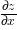and the second those of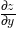.
class matplotlib.tri.CubicTriInterpolator(triangulation, z, kind='min_E', trifinder=None, dz=None)

Bases: matplotlib.tri.triinterpolate.TriInterpolator

A CubicTriInterpolator performs cubic interpolation on triangular grids.

In one-dimension - on a segment - a cubic interpolating function is defined by the values of the function and its derivative at both ends. This is almost the same in 2-d inside a triangle, except that the values of the function and its 2 derivatives have to be defined at each triangle node.

The CubicTriInterpolator takes the value of the function at each node - provided by the user - and internally computes the value of the derivatives, resulting in a smooth interpolation. (As a special feature, the user can also impose the value of the derivatives at each node, but this is not supposed to be the common usage.)

Parameters : triangulation : Triangulation object The triangulation to interpolate over. z : array_like of shape (npoints,) Array of values, defined at grid points, to interpolate between. kind : {‘min_E’, ‘geom’, ‘user’}, optional Choice of the smoothing algorithm, in order to compute the interpolant derivatives (defaults to ‘min_E’): if ‘min_E’: (default) The derivatives at each node is computed to minimize a bending energy. if ‘geom’: The derivatives at each node is computed as a weighted average of relevant triangle normals. To be used for speed optimization (large grids). if ‘user’: The user provides the argument dz, no computation is hence needed. trifinder : TriFinder object, optional If not specified, the Triangulation’s default TriFinder will be used by calling matplotlib.tri.Triangulation.get_trifinder(). dz : tuple of array_likes (dzdx, dzdy), optional Used only if kind =’user’. In this case dz must be provided as (dzdx, dzdy) where dzdx, dzdy are arrays of the same shape as z and are the interpolant first derivatives at the triangulation points.

Notes

This note is a bit technical and details the way a CubicTriInterpolator computes a cubic interpolation.

The interpolation is based on a Clough-Tocher subdivision scheme of the triangulation mesh (to make it clearer, each triangle of the grid will be divided in 3 child-triangles, and on each child triangle the interpolated function is a cubic polynomial of the 2 coordinates). This technique originates from FEM (Finite Element Method) analysis; the element used is a reduced Hsieh-Clough-Tocher (HCT) element. Its shape functions are described in [R1]. The assembled function is guaranteed to be C1-smooth, i.e. it is continuous and its first derivatives are also continuous (this is easy to show inside the triangles but is also true when crossing the edges).

In the default case (kind =’min_E’), the interpolant minimizes a curvature energy on the functional space generated by the HCT element shape functions - with imposed values but arbitrary derivatives at each node. The minimized functional is the integral of the so-called total curvature (implementation based on an algorithm from [R2] - PCG sparse solver):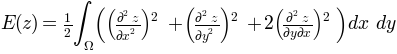If the case kind =’geom’ is chosen by the user, a simple geometric approximation is used (weighted average of the triangle normal vectors), which could improve speed on very large grids.

References

 [R1] (1, 2) Michel Bernadou, Kamal Hassan, “Basis functions for general Hsieh-Clough-Tocher triangles, complete or reduced.”, International Journal for Numerical Methods in Engineering, 17(5):784 - 789. 2.01.
 [R2] (1, 2) C.T. Kelley, “Iterative Methods for Optimization”.

Methods

 __call__ (x, y) Returns interpolated values at x,y points gradient (x, y) Returns interpolated derivatives at x,y points
__call__(x, y)

Returns a masked array containing interpolated values at the specified x,y points.

Parameters : x, y : array-like x and y coordinates of the same shape and any number of dimensions. z : np.ma.array Masked array of the same shape as x and y ; values corresponding to (x, y) points outside of the triangulation are masked out.

Returns a list of 2 masked arrays containing interpolated derivatives at the specified x,y points.

Parameters : x, y : array-like x and y coordinates of the same shape and any number of dimensions. dzdx, dzdy : np.ma.array 2 masked arrays of the same shape as x and y ; values corresponding to (x,y) points outside of the triangulation are masked out. The first returned array contains the values ofand the second those of.

Examples

An example of effective application is shown below (plot of the direction of the vector field derivated from a known potential field):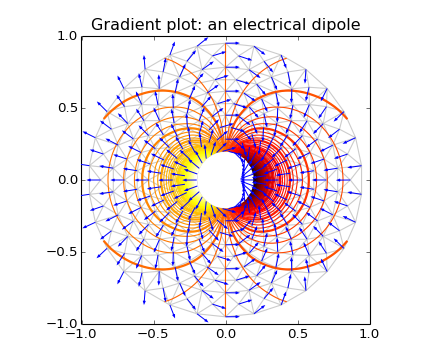class matplotlib.tri.TriRefiner(triangulation)

Abstract base class for classes implementing mesh refinement.

A TriRefiner encapsulates a Triangulation object and provides tools for mesh refinement and interpolation.

Derived classes must implements:

• refine_triangulation(return_tri_index=False, **kwargs) , where the optional keyword arguments kwargs are defined in each TriRefiner concrete implementation, and which returns :

• a refined triangulation
• optionally (depending on return_tri_index), for each point of the refined triangulation: the index of the initial triangulation triangle to which it belongs.
• refine_field(z, triinterpolator=None, **kwargs) , where:

• z array of field values (to refine) defined at the base triangulation nodes
• triinterpolator is a TriInterpolator (optional)
• the other optional keyword arguments kwargs are defined in each TriRefiner concrete implementation

and which returns (as a tuple) a refined triangular mesh and the interpolated values of the field at the refined triangulation nodes.

class matplotlib.tri.UniformTriRefiner(triangulation)

Bases: matplotlib.tri.trirefine.TriRefiner

Uniform mesh refinement by recursive subdivisions.

Parameters : triangulation : Triangulation The encapsulated triangulation (to be refined)
refine_field(z, triinterpolator=None, subdiv=3)

Refines a field defined on the encapsulated triangulation.

Returns refi_tri (refined triangulation), refi_z (interpolated values of the field at the node of the refined triangulation).

Parameters : z : 1d-array-like of length n_points Values of the field to refine, defined at the nodes of the encapsulated triangulation. (n_points is the number of points in the initial triangulation) triinterpolator : TriInterpolator, optional Interpolator used for field interpolation. If not specified, a CubicTriInterpolator will be used. subdiv : integer, optional Recursion level for the subdivision. Defaults to 3. Each triangle will be divided into 4**subdiv child triangles. refi_tri : Triangulation object The returned refined triangulation refi_z : 1d array of length: refi_tri node count. The returned interpolated field (at refi_tri nodes)

Examples

The main application of this method is to plot high-quality iso-contours on a coarse triangular grid (e.g., triangulation built from relatively sparse test data):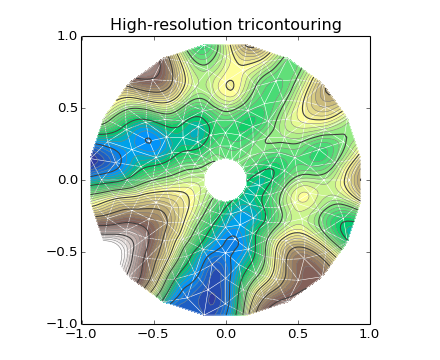refine_triangulation(return_tri_index=False, subdiv=3)

Computes an uniformly refined triangulation refi_triangulation of the encapsulated triangulation.

This function refines the encapsulated triangulation by splitting each father triangle into 4 child sub-triangles built on the edges midside nodes, recursively (level of recursion subdiv). In the end, each triangle is hence divided into 4**subdiv child triangles. The default value for subdiv is 3 resulting in 64 refined subtriangles for each triangle of the initial triangulation.

Parameters : return_tri_index : boolean, optional Boolean indicating whether an index table indicating the father triangle index of each point will be returned. Default value False. subdiv : integer, optional Recursion level for the subdivision. Defaults value 3. Each triangle will be divided into 4**subdiv child triangles. refi_triangulation : Triangulation The returned refined triangulation found_index : array-like of integers Index of the initial triangulation containing triangle, for each point of refi_triangulation. Returned only if return_tri_index is set to True.
class matplotlib.tri.TriAnalyzer(triangulation)

Define basic tools for triangular mesh analysis and improvement.

A TriAnalizer encapsulates a Triangulation object and provides basic tools for mesh analysis and mesh improvement.

Parameters : triangulation : Triangulation object The encapsulated triangulation to analyze.

Attributes

 scale_factors Factors to rescale the triangulation into a unit square.
circle_ratios(rescale=True)

Returns a measure of the triangulation triangles flatness.

The ratio of the incircle radius over the circumcircle radius is a widely used indicator of a triangle flatness. It is always <= 0.5 and == 0.5 only for equilateral triangles. Circle ratios below 0.01 denote very flat triangles.

To avoid unduly low values due to a difference of scale between the 2 axis, the triangular mesh can first be rescaled to fit inside a unit square with scale_factors (Only if rescale is True, which is its default value).

Parameters : rescale : boolean, optional If True, a rescaling will be internally performed (based on scale_factors, so that the (unmasked) triangles fit exactly inside a unit square mesh. Default is True. circle_ratios : masked array Ratio of the incircle radius over the circumcircle radius, for each ‘rescaled’ triangle of the encapsulated triangulation. Values corresponding to masked triangles are masked out.

Eliminates excessively flat border triangles from the triangulation.

Returns a mask new_mask which allows to clean the encapsulated triangulation from its border-located flat triangles (according to their circle_ratios()). This mask is meant to be subsequently applied to the triangulation using matplotlib.tri.Triangulation.set_mask() . new_mask is an extension of the initial triangulation mask in the sense that an initially masked triangle will remain masked.

The new_mask array is computed recursively ; at each step flat triangles are removed only if they share a side with the current mesh border. Thus no new holes in the triangulated domain will be created.

Parameters : min_circle_ratio : float, optional Border triangles with incircle/circumcircle radii ratio r/R will be removed if r/R < min_circle_ratio. Default value: 0.01 rescale : boolean, optional If True, a rescaling will first be internally performed (based on scale_factors ), so that the (unmasked) triangles fit exactly inside a unit square mesh. This rescaling accounts for the difference of scale which might exist between the 2 axis. Default (and recommended) value is True. new_mask : array-like of booleans Mask to apply to encapsulated triangulation. All the initially masked triangles remain masked in the new_mask.

Notes

The rationale behind this function is that a Delaunay triangulation - of an unstructured set of points - sometimes contains almost flat triangles at its border, leading to artifacts in plots (especially for high-resolution contouring). Masked with computed new_mask, the encapsulated triangulation would contain no more unmasked border triangles with a circle ratio below min_circle_ratio, thus improving the mesh quality for subsequent plots or interpolation.

Examples

Please refer to the following illustrating example: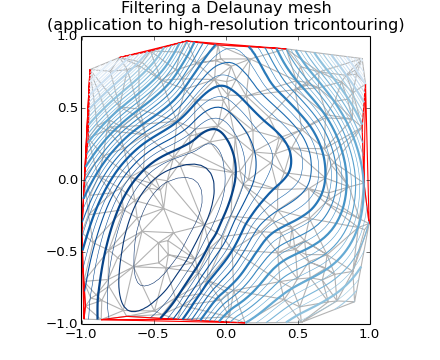scale_factors

Factors to rescale the triangulation into a unit square.

Returns k, tuple of 2 scale factors.

Returns : k : tuple of 2 floats (kx, ky) Tuple of floats that would rescale the triangulation : [triangulation.x * kx, triangulation.y * ky] fits exactly inside a unit square.